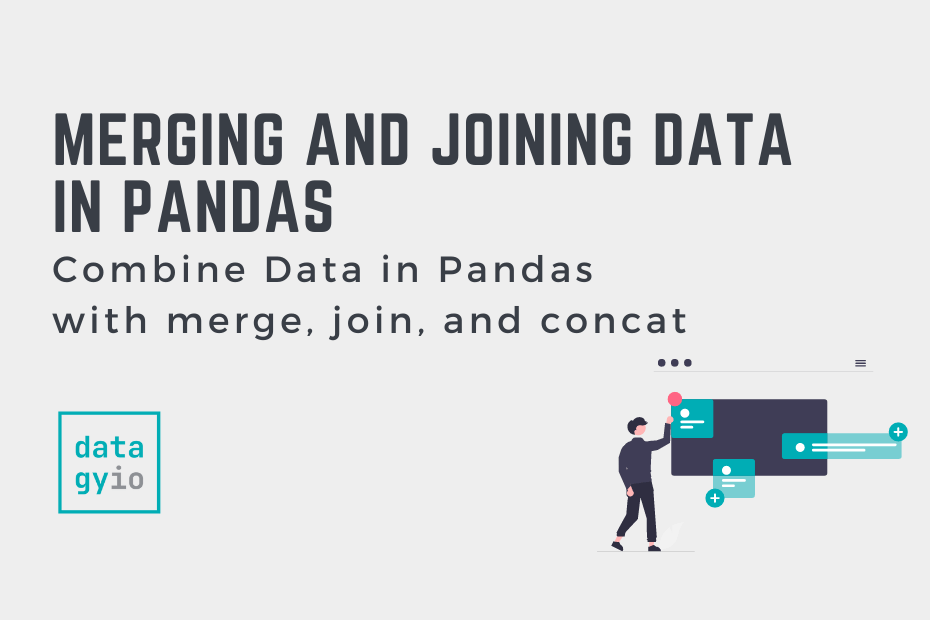# 30 Days of Python## Combine Data in Pandas with merge, join, and concat

In this tutorial, you’ll learn how to combine data in Pandas by merging, joining, and concatenating DataFrames. You’ll learn how to perform database-style merging of DataFrames based on common columns or indices using the merge() function and the .join() method.… Read More »Combine Data in Pandas with merge, join, and concat## Introduction to Machine Learning in Python

In this tutorial, you’ll gain an understanding of what machine learning is and how Python can help you take on machine learning projects. Understanding what machine learning is, allows you to understand and see its pervasiveness. In many cases, people… Read More »Introduction to Machine Learning in Python## Introduction to Scikit-Learn (sklearn) in Python

In this tutorial, you’ll learn what Scikit-Learn is, how it’s used, and what its basic terminology is. While Scikit-learn is just one of several machine learning libraries available in Python, it is one of the best known. The library provides… Read More »Introduction to Scikit-Learn (sklearn) in Python## Splitting Your Dataset with Scitkit-Learn train_test_split

In this tutorial, you’ll learn how to split your Python dataset using Scikit-Learn’s train_test_split function. You’ll gain a strong understanding of the importance of splitting your data for machine learning to avoid underfitting or overfitting your models. You’ll also learn… Read More »Splitting Your Dataset with Scitkit-Learn train_test_split## Linear Regression in Scikit-Learn (sklearn): An Introduction

In this tutorial, you’ll learn how to learn the fundamentals of linear regression in Scikit-Learn. Throughout this tutorial, you’ll use an insurance dataset to predict the insurance charges that a client will accumulate, based on a number of different factors. You’ll… Read More »Linear Regression in Scikit-Learn (sklearn): An Introduction## Introduction to Random Forests in Scikit-Learn (sklearn)

In this tutorial, you’ll learn what random forests in Scikit-Learn are and how they can be used to classify data. Decision trees can be incredibly helpful and intuitive ways to classify data. However, they can also be prone to overfitting,… Read More »Introduction to Random Forests in Scikit-Learn (sklearn)## Introduction to Pandas for Data Science

In this tutorial, you’ll learn how to dive into the wonderful world of Pandas. Pandas is a Python package that provides fast and flexible data structures used for data manipulation and analysis. By the end of this tutorial, you’ll have… Read More »Introduction to Pandas for Data Science## Indexing, Selecting, and Assigning Data in Pandas

In this tutorial, you’ll learn how to index, select and assign data in a Pandas DataFrame. Understanding how to index and select data is an important first step in almost any exploratory work you’ll take on in data science. Similarly,… Read More »Indexing, Selecting, and Assigning Data in Pandas## Summarizing and Analyzing a Pandas DataFrame

In this tutorial, you’ll learn how to quickly summarize and analyze a Pandas DataFrame. By the end of this tutorial, you’ll have learned to take on some exploratory analysis of your dataset using pandas. You’ll learn how to calculate general… Read More »Summarizing and Analyzing a Pandas DataFrame## Transforming Pandas Columns with map and apply

In this tutorial, you’ll learn how to transform your Pandas DataFrame columns using vectorized functions and custom functions using the map and apply methods. By the end of this tutorial, you’ll have a strong understanding of how Pandas applies vectorized… Read More »Transforming Pandas Columns with map and apply## NumPy for Data Science in Python

In this tutorial, you’ll learn how to use Python’s NumPy library for data science. You’ll learn why the library matters in the realm of data science and how it’s foundational for many other libraries. You’ll learn about the NumPy ndarray… Read More »NumPy for Data Science in Python## Python 3: Installation and Setup

Installing or upgrading Python is an important step in becoming a Python programmer. There are a number of different ways that you can install and upgrade Python. This tutorial will cover off using the official installer from Python.org and the… Read More »Python 3: Installation and Setup